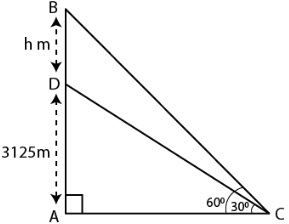Guru

# An aeroplane when flying at a heigt of 3125 m from the ground passes vertically below another plane at an instant when the angles of elevation of the two planes from the same point on the ground are 300 and 600 respectively. Find the distance between the two planes at the instant.

• 0

sir this is the question from the book -ML aggarwal( avichal publication) class 10th , chapter20 , heights and distances
we have the information that An aeroplane when flying at a heigt of 3125 m from the ground passes vertically below another plane at an instant when the angles of elevation of the two planes from the same point on the ground are 300 and 600 respectively.
we are asked to Find the distance between the two planes at the instant.

question no 16 , heights and distances , ICSE board

Share

1. Consider the distance between two planes = h m

It is given that

AD = 3125 m, ∠ACB = 600 and ∠ACD = 300In triangle ACD

Substituting the values

1/√3 = 3125/AC

AC = 3125√3 ……. (1)

In triangle ABC

tan 600 = AB/AC

Substituting the values

√3 = (AD + DB)/ AC

So we get

√3 = (3125 + h)/ AC

AC = (3125 + h)/ √3 ….. (2)

Using both the equations

(3125 + h)/ √3 = 3125√3

By further calculation

h = (3125√3 × √3) – 3125

h = 3125 × 3 – 3125

h = 9375 – 3125

h = 6250 m

Therefore, the distance between two planes at the instant is 6250 m.

• 1